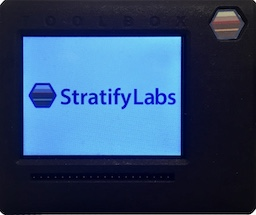# Low Pass Filters with Microcontroller ADCs###### Introducing the Stratify Toolbox

Are you a printf()-er or a code-stepper? The Stratify Toolbox is a printf()-ing awesome debug tool!A filter is a useful tool for removing unwanted signals from a sensor measurement. There is complicated math behind how they work, but you just need to know a simple formula to use them in many embedded designs.

Filters operate on frequencies and comes in three basic varieties: low pass, high pass, and band (middle) pass. A low pass filter does exactly what the name implies: it passes low frequencies and rejects higher ones. Low pass filters are broadly characterized by a cutoff frequency that defines which frequencies are passed and which are blocked. More accurately, low pass filters pass low frequencies and attenuate higher ones. The higher the frequency is above the cutoff, the more it is attenuated. Again, there is complicated math that explains how and why this works, but we just need to worry about a simple formula defining the cutoff frequency.

A low pass filter can be as simple as a resistor and a capacitor (shown above). This circuit’s cutoff frequency in radians is omega=1/(RC). To convert to hertz, we use omega = 2 pi f so the cutoff frequency becomes f=1/(2 pi RC).

As an example, let’s assume the above circuit is used to measure a battery voltage. Because battery voltage changes very slowly, we can use a low pass filter with a sub 10Hz cutoff frequency to measure the battery voltage and reject higher frequency noise.

When selecting R and C, we want to ensure R is low enough to allow a sufficient current to drive the ADC but not so low that C must be impractically large to get the desired cutoff frequency. A 10K resistor with a 10uF capacitor give a cutoff frequency of 1.59Hz which would work well when measuring battery voltage.Another example is measuring the voice input from a microphone. Voice commonly occupies the 0 to 4000Hz range of frequencies. In order to capture 4000Hz on the ADC, the signal will need to be sampled at 8000Hz (see Nyquist Frequency). However, we design the LPF to have a cutoff frequency of 4000Hz. This ratio generally applies to all signals: the LPF cutoff frequency should be set to half the sampling frequency.

Just remember the simple equation omega=1/(RC), and you can use LPFs to reduce noise when measuring signals on any ADC input.

X